Home > Application Notes > Time Lag of Pressure Systems

# Time Lag of Pressure Systems

When a pressure system is measuring varying or changing pressures, the pressures displayed by the pressure transducer/manometer may not be correct. This effect is typically considered to consist of two components:

• Acoustic lag: pressure disturbances propagate at the speed of sound. Thus it takes a finite time interval for a pressure disturbance or change to be transmitted down the connecting tubing to the pressure sensor.
• Pressure lag: due to viscosity in the tubes, it takes a finite time for the magnitude of the pressure change to register.

For most systems of practical interest, acoustic lag is typically small. In the following presentation, data will be presented to convey the effect of tubing properties on the time lag of the system. The presented data is intended to be illustrative only and should not be used for numerical reference. Almost no manometers are capable of discerning rapidly changing pressures, due to the large lengths of tubing attached to these instruments, as well as their internal mechanical and electrical design. However, it is useful to have an understanding of parameters effecting the lag of measured pressures when making measurements, so as to minimize errors.

Suppose you wish to measure the pressure in a duct, which for example may be 3kPa. After connecting your tubing to the duct, it takes a finite amount of time for the pressure at the face of the transducer in the manometer to reach 3kPa, i.e. there is a lag. Numerous studies have been conducted to determine the dependence of this lag on instrument and tubing parameters, primarily for systems where real time measurements are needed, such as on aircraft. Sinclair and Robbins (Sinclair, A. R. and Robins, A. W., Method for the Determination of the Time Lag in Pressure Measuring Systems Incorporating Capillaries," NACA TN 2793, Sept. 1952) developed a theory to estimate the time lag in tubing systems. For a single tube, they suggest the following equation to describe the lag: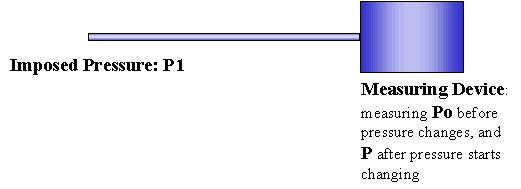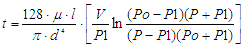(1)

where

P= pressure at any time t

Po = initial pressure at time to (at the transducer)

P1 = final applied pressure at orifice or open end of tubing

V = total measuring system volume

l = length of tubing

d = diameter of tubing

μ = viscosity

t = time, seconds

This equation is only valid for over damped systems, such that the pressure signal is always attenuated and never amplified. The criterion which needs to be satisfied for an over damped system is: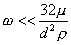(2)

where

ρ = fluid density

ω = frequency of changing imposed pressure signal

As may have been surmised, the lag of a pressure system is dependent on the length of the tube, its diameter, and the pressure differential imposed across the system. A "feel" for the dependence of the lag of a pressure measurement system may be gained from the figures below.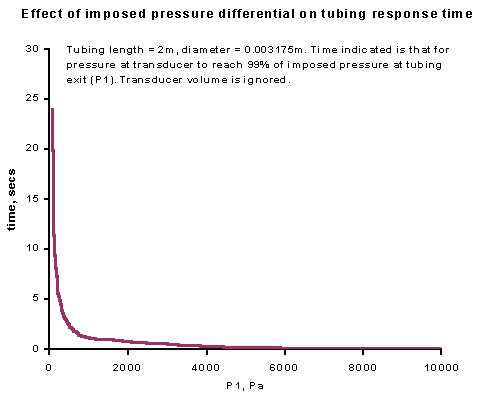Fig. 1 Effect of imposed pressure differential on tubing response time

Figure 1 shows the calculated delay, using Eq. (1) for a fixed set of tubing dimensions, but with different pressure differentials across the system. These may be interpreted as how long it takes for the pressure at the manometer side of the tubing to reach that applied at the other end, when the difference between the two is, essentially, P1. As the figure shows, increasing pressure differentials rapidly decrease the time lag of the system, while measuring low pressure, can result in fairly long time lags.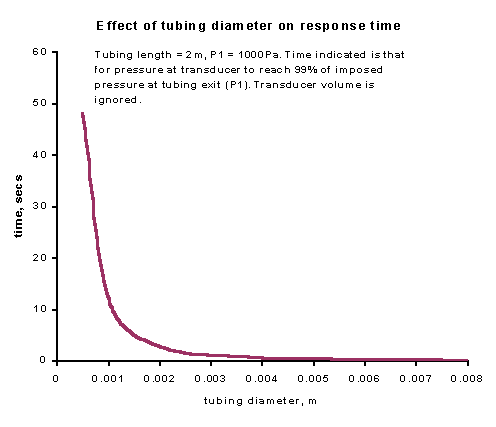Fig. 2 Effect of tubing diameter on response time

Figure 2 presents the effect of tubing diameter, with other variables fixed. Tubing diameter is seen to have a profound effect on the lag time, especially for small diameter tubing. However, increasing the tubing diameter beyond approximately 3mm, has little impact, and as mentioned, may violate the applicability of Eq. (1) (see Eq. (2)) for analysis depending on the rapidity (or frequency) with which the pressure signal changes.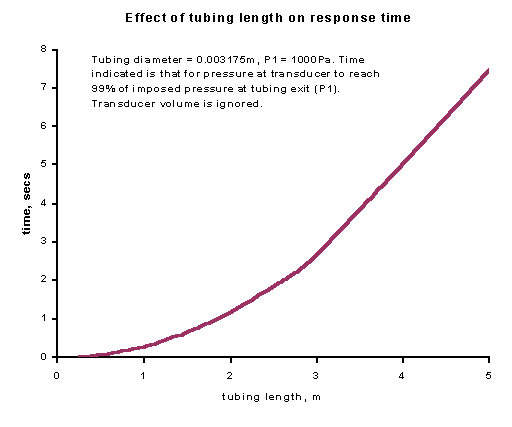Fig. 3 Effect of tubing length response time

Figure 3 displays the effect of tubing length on the lag time. As may be seen, increasing tubing length is associated with an increase in lag time. However, as may be seen, the dependency of lag time on tubing length is not as sensitive as it is to pressure differential or tubing diameter.

Although the data may suggest that it would be optimal to have as large a tube diameter as possible, this may cause the system to no longer behave as an over damped one, with possible resonance (depending on the frequency of the signal fluctuations) and hence large signal gain affecting the results.

Email:
inform@flowkinetics.com

Tel: (979) 680-0659

Tel: (888) 670-1927

Fax: (979) 680-0659